# Resources tagged with: Graph sketching

Filter by: Content type:
Age range:
Challenge level:

### There are 17 results

Broad Topics > Coordinates, Functions and Graphs > Graph sketching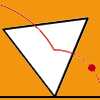### Speeding Up, Slowing Down

##### Age 11 to 14Challenge Level

Experiment with the interactivity of "rolling" regular polygons, and explore how the different positions of the red dot affects its speed at each stage.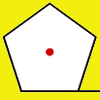### How Far Does it Move?

##### Age 11 to 14Challenge Level

Experiment with the interactivity of "rolling" regular polygons, and explore how the different positions of the red dot affects the distance it travels at each stage.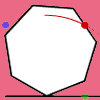### Up and Across

##### Age 11 to 14Challenge Level

Experiment with the interactivity of "rolling" regular polygons, and explore how the different positions of the dot affects its vertical and horizontal movement at each stage.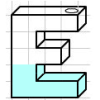### Mathsjam Jars

##### Age 14 to 16Challenge Level

Imagine different shaped vessels being filled. Can you work out what the graphs of the water level should look like?### Exploring Cubic Functions

##### Age 14 to 18Challenge Level

Quadratic graphs are very familiar, but what patterns can you explore with cubics?### Curve Fitter

##### Age 14 to 18Challenge Level

This problem challenges you to find cubic equations which satisfy different conditions.### Bio Graphs

##### Age 14 to 16Challenge Level

What biological growth processes can you fit to these graphs?### Maths Filler 2

##### Age 14 to 16Challenge Level

Can you draw the height-time chart as this complicated vessel fills with water?### More Parabolic Patterns

##### Age 14 to 18Challenge Level

The illustration shows the graphs of twelve functions. Three of them have equations y=x^2, x=y^2 and x=-y^2+2. Find the equations of all the other graphs.### Parabolic Patterns

##### Age 14 to 18Challenge Level

The illustration shows the graphs of fifteen functions. Two of them have equations y=x^2 and y=-(x-4)^2. Find the equations of all the other graphs.### Back Fitter

##### Age 14 to 18Challenge Level

10 graphs of experimental data are given. Can you use a spreadsheet to find algebraic graphs which match them closely, and thus discover the formulae most likely to govern the underlying processes?### Immersion

##### Age 14 to 16Challenge Level

Various solids are lowered into a beaker of water. How does the water level rise in each case?### Guessing the Graph

##### Age 14 to 16Challenge Level

Can you suggest a curve to fit some experimental data? Can you work out where the data might have come from?### Curve Hunter

##### Age 14 to 18Challenge Level

This problem challenges you to sketch curves with different properties.### What's That Graph?

##### Age 14 to 18Challenge Level

Can you work out which processes are represented by the graphs?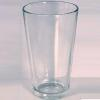### Fill Me Up

##### Age 11 to 14Challenge Level

Can you sketch graphs to show how the height of water changes in different containers as they are filled?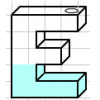### Maths Filler

##### Age 11 to 14Challenge Level

Imagine different shaped vessels being filled. Can you work out what the graphs of the water level should look like?